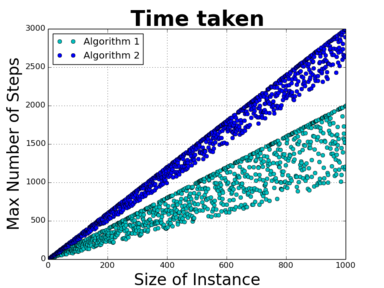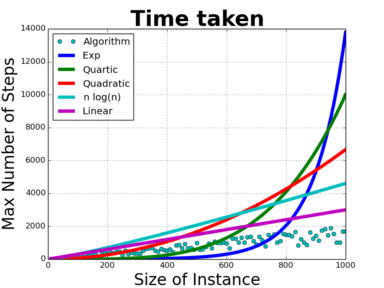# Introducing Big Oh

 Recent changes Table of contents
 Links to this page FRONT PAGE / INDEXSubscribe!My latest posts can be found here:
Previous blog posts:
Additionally, some earlier writings:

## What is "Big Oh"?

So a brief recap:

• A Problem is a(n infinite) collection of Instances;
• An Instance is a "Challenge/Response" pair;
• An Algorithm, when given a Challenge, returns the Response;
• An Instance has a concept of its Size;
• For each Size of Instance, we look at the worst case time taken by an Algorithm;
• We chart that maximum time as a function of Instance Size;
• We look at how that "maximum time taken" grows.

Last time I said:

 If you do this for two different algorithms and the results are, apart from a scaling factor, pretty much the same, then the algorithms are said to have the same "Time Complexity".

This post is going to look at that carefully, because while it's true that if the "time taken" functions of two algorithms "look like" each other in the long run then they have the same time complexity, that's not enough. Algorithms can behave erratically, so the concept of "this looks like that" isn't really enough, we would like to do better.

 This post is deliberately gentle and repeats itself a lot. The ideas in here are important, and can take some time to assimilate. There is also, as a work in progress, and "Autobahn" version of these posts, a high-speed romp through the basic ideas. I'll link to that soon, and replace the content of this aside with a pointer.
So we will look at how much better we can do. In this post we will look at "Big Oh" notation. I'm expecting that people will mostly skim this, get the broad sweep of ideas, and then come back to it later to check on the details (if they care).

So don't be too worried if some of the details don't make sense yet. The broad conclusion is this:

 "Big Oh" notation gives us a way of understanding that in the long run, one algorithm can be better than another, and gives us a way to quantify that.

## Not really linear, but sort of.Fig 1: Are these "Linear"?
So while the statement from last time isn't technically true, it does give the right intuition. The problem with intuition is that it can let you down in cases where we see "pathological behaviour". Most of the time it doesn't matter, but it's nice to have definitions that cover all cases, so we don't have to worry about exceptions.

So let's have a look at the time-performance of two hypothetical algorithms on the same problem. They are different algorithms, and are quite complex, so their behaviours are not especially easy to understand. Even so, let's posit that they behave as shown in this chart.

These two algorithms behave somewhat erratically, with some instance sizes taking much less than others, but broadly, both algorithms take time that grows linearly(ish) with the size of the instance. In some sense these two algorithms are behaving very similarly, and it would be great to have some way of capturing that mathematically.

Then we remember two things:

• Firstly, we really only care about how an algorithm behaves at worst, and ...
• Secondly, we really only care about how it behaves in the long run.

So what we look for is a function that, in the long run, is larger than the "run time" for our algorithm. That gives us an upper bound.

Well in this case that's easy, we can just use $2^n$. That will certainly exceed our algorithm's run time.

Hmm. Not really either helpful or informative. What we really want is the best upper bound.

So here's what has been proven to be both useful and informative.

## Getting an upper bound with "Domination"

The concept we use is to think of one function "dominating" another. In formulas, we say that function $f(n)$ "dominates" function $g(n)$ if, after some time, $f(n)$ is always bigger than $g(n).$

OK, so that's not quite true. We allow for a constant, and we say something more precise about "in the long run" and we get this:

 Function $f(n)$ dominates function $g(n)$ if there's a constant $c$ and a number $N$ such that whenever $n>N$ we have $c{\cdot}f(n) > |g(n)|$

 Yes, I am literally saying the same thing, but using different words. This is an important concept, and when you think about the exact details, it's not entirely obvious.
In other words, $f$ dominates $g$ if, in the long run, $f$ is always bigger than $g$ (up to a constant).

This isn't quite enough ...

Suppose by way of an example that the time taken by algorithm $A$ is linear, so we can write $T_A(n)=kn$ for some constant $k$. Using our definition, it is true to say that this is dominated by $n^2,$ because it doesn't matter what $k$ might be, eventually $n^2$ will be bigger than $k\cdot{n}$. But that isn't really very helpful, and isn't as informative as we might like. By itself the definition of "to dominate" doesn't really capture how the algorithm behaves. In a sense it's not aggressive enough, it's too "loose".

But an upper bound is an upper bound, and what we're really asking for is for the best known upper bound. That brings us to our final form.

So the set-up is this. We have a problem, $P$, and for that problem we have an algorithm, $A$. At best we can we compute an upper bound for how long $A$ takes for each size of instance of $P$. We'll call that $T_A(n).$ For simple problems we might be able to compute that exactly, but for sufficiently complex problems we can usually only get an upper bound.

Then we say this:

• Algorithm $A$ is, at worst, of complexity $C$, if $C$ dominates $T_A$.Comparing growth rates

## It's actually more complicated than that

### (now there's a surprise)

So look again at the plot for our hypothetical algorithm, and overlay various functions that increase as $n$ increases.

It's not helpful, I know, but it's true to say that the time function for this algorithm is dominated by $2^n$. It's substantially better to say that it's dominated by $n^4$, and it's better again to say it's dominated by $n^2$. Better still, it's dominated by $n\cdot\log(n)$.

But it's also dominated by the function $f(n)=n$, and by $g(n)=n+5,$ and perhaps more surprisingly, by $h(n)=n-\log(n).$

What?

Yes, believe it or not the function $f(n)=n$ is dominated by $n-\log(n)$. To see this, we look at the definition. That asks if there is a $k$ and an $N$ such that when $n>N$ we have $k(n-\log(n))>n.$ Rearranging that, we want to know if there is a $k$ such that when $n$ is big enough, $(k-1)n>k\log(n).$

But just taking $k=2$ suffices, so yes, $n$ is dominated by $n-\log(n)$.

You might think that would render the whole concept sufficiently non-intuitive as to be useless, but actually it lets us find the idea of the "dominating term".

In essence, $n$ grows faster than $\log(n)$, so including any terms that are essentially just $\log(n)$ doesn't change things. It's the fastest growing term that matters, the slower growing don't change things enough to make a difference.

 Oh, and by the way ... yes, the algorithms shown in Fig 1 are "linear".
We need to make that more precise, but before we can do so we need some new notation, and a few fundamental ideas about relationships between things.

 <<<< Prev <<<< Constant Differences : >>>> Next >>>> Mathematical Relations ...You can follow me on Mathstodon.

 Of course, you can alsofollow me on twitter:## Send us a comment ...

 You can send us a message here. It doesn't get published, it just sends us an email, and is an easy way to ask any questions, or make any comments, without having to send a separate email. So just fill in the boxes and then

 Your name : Email : Message :

# Links on this page

 Site hosted by Colin and Rachel Wright: Maths, Design, Juggling, Computing, Embroidery, Proof-reading, and other clever stuff.Suggest a change ( <-- What does this mean?) / Send me email
Front Page / All pages by date / Site overview / Top of page